# NCERT Solutions for Class 7 Maths Chapter 2 Fractions and Decimals Ex 2.4

NCERT Solutions for Class 7 Maths Chapter 2 Fractions and Decimals Ex 2.4

### NCERT Solutions for Class 7 Maths Chapter 2 Fractions and Decimals Ex 2.4

NCERT Solutions for Class 7 Maths Chapter 2 Fractions and Decimals Exercise 2.4

Ex 2.4 Class 7 Maths Question 1.
Find:Solution:Ex 2.4 Class 7 Maths Question 2.
Find the reciprocal of each of the following fractions. Classify the reciprocals as proper fractions, improper fractions and whole numbers.Ex 2.4 Class 7 Maths Question 3.
Find: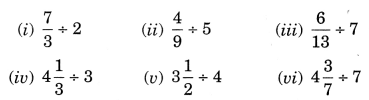Solution: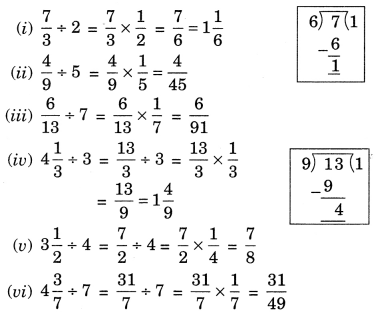Ex 2.4 Class 7 Maths Question 4.
Find: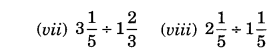Solution: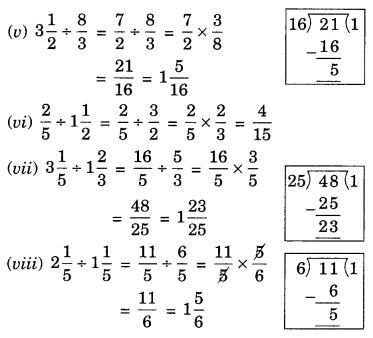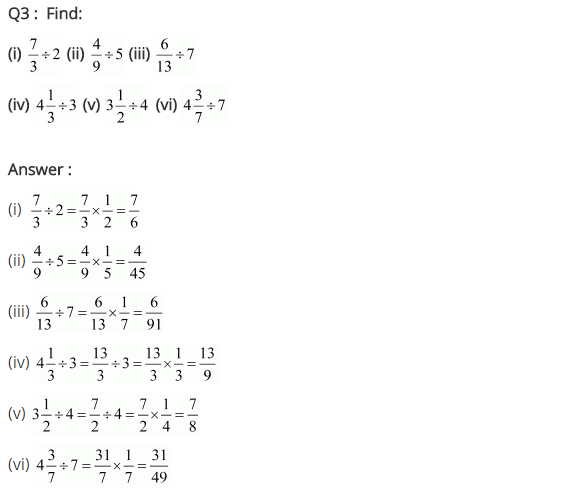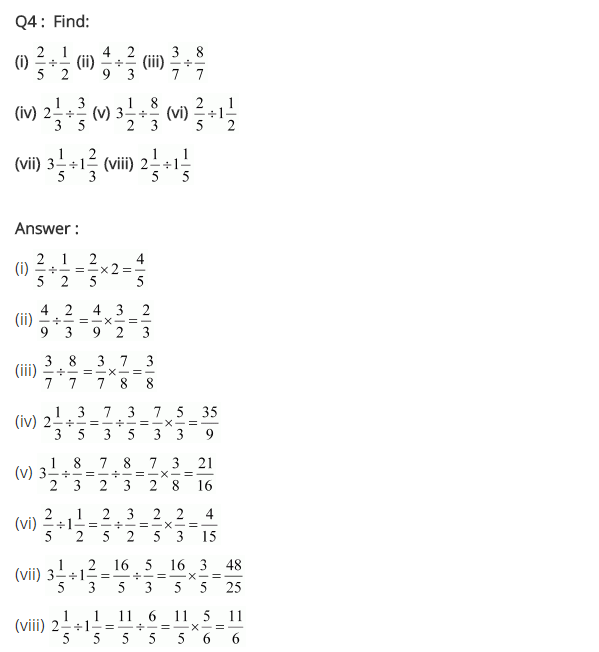## SabDekho

The Complete Educational Website